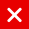# IOTA Tutorial

## Merkle Tree

In this video series different topics will be explained which will help you to understand IOTA. It is recommended to watch each video sequentially as I may refer to certain IOTA topics explained earlier.

The main objective of this video is to provide you with some basic knowledge about the Merkle tree. The Merkle tree is used in IOTA’s Masked Authenticated Messaging. IOTA’s Masked Authenticated Messaging will be explained in IOTA tutorial 19.

A hash tree or Merkle tree is a tree structure in which each leaf node is a hash of a block of data and each non-leaf node is a hash of its children. This results in a single hash called the Merkle root. If every node has two children, the tree is called a binary hash tree.

Why use a Merkle tree?

Why not hash all messages (m0-m15), append the hashed messages and then hash it all to get one root hash value.

Bob get the root hash from a trusted source.

If Alice wants to proof to Bob that m6 is not tampered with, she needs to send message m6 and all other hashed values:

H(m0), H(m1), H(m2), H(m3), H(m4), H(m5), H(m7), H(m8), H(m9), H(m10), H(m11), H(m12), H(m13), H(m14) and H(m15) to Bob.

Bob hashes message m6, append all hashes to a single string and hash this string to get one root hash. Bob compares this new root hash with the trusted source root hash to check if message m6 is not tampered with.

In this example Alice has to provide 15 hashed values and the message m6 to Bob to prove that message m6 is not tampered with. A much better solution is using a Merkle tree (16 leaves) Again as before Bob gets the root hash from a trusted source. If Alice wants to proof that m6 is not tampered with, she needs to send m6 and 4 hashed values to Bob. With the received information Bob calculates the root hash value. Bob compares this root hash with the trusted source root hash to check if message m6 is not tampered with. In this example Alice only needs to provide 4 hashed values and the message m6 to Bob to prove that message m6 is not tampered with.

Using a Merkle tree provides integrity and validity of your data using a small amount of data that a trusted authority has to maintain. This also means little memory / disk space is needed.

If a Merkle tree has more leaves less hashed values are needed, in comparison to the number of leaves, to validate if a message is not tampered with.

A perfect Merkle binary tree has the following properties:

• The number of leaves is always 2n (n=0, 1, 2, 3,..).
• Each node has 0 or 2 children.
• All leaves are on the same level. In a perfect binary tree the following formulas can be applied:
• Total number of leaves = L = (N + 1) / 2
• Total number of nodes = N = 2L – 1
• Total number of levels = LV = log2(L) + 1 = (ln(L) / ln(2)) + 1
• Number of leaves = L = 1
• Number of nodes = N = 1
• Number of levels = LV = 1
• Number of leaves = L = 2
• Number of nodes = N = 3
• Number of levels = LV = 2
• Number of leaves = L = 4
• Number of nodes = N = 7
• Number of levels = LV = 3
• Number of leaves = L = 8
• Number of nodes = N = 15
• Number of levels = LV = 4
• Number of leaves = L = 16
• Number of nodes = N = 31
• Number of levels = LV = 5
You might also like

This website uses cookies to improve your experience. We'll assume you're ok with this, but you can opt-out if you wish. AcceptRead More

Did you know?

Buy in without the stress of trying to time the market

when you setup a savings plan. If you haven’t started a plan yet, head to your Bitpanda account to get started!

Purchasing cryptocurrencies comes with a number of risks.
Never invest money that you can't afford to lose.You can automatically invest

in IOTA with Bitpanda Savings!